# Union of sets

(diff) ← Older revision | Latest revision (diff) | Newer revision → (diff)

sum of sets

One of the basic operations on (collections of) sets. Suppose one has some (finite or infinite) collectionof sets. Then the collection of all elements that belong to at least one of the sets in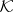is called the union, or, more rarely, the sum, of (the sets in); it is denoted by.

In case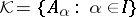, the union is also denoted by,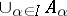,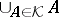, or, more rarely, by.
If the sets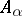are disjoint, then in the category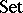the union of the objectsis the sum of these objects in the categorical sense. In general, the sum of objects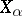is the disjoint union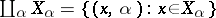. The natural imbeddings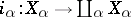are given by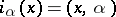. Thus,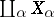together with the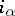,, satisfies the universal property for categorical sums: For every family of mappings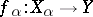there is a unique mapping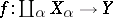such that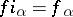.